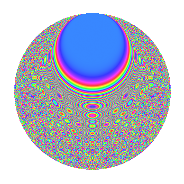# Properties

 Label 3234.2.rLevel 3234 Weight 2 Character orbit r Rep. character $$\chi_{3234}(463,\cdot)$$ Character field $$\Q(\zeta_{7})$$ Dimension 576 Sturm bound 1344

# Related objects

## Defining parameters

 Level: $$N$$ $$=$$ $$3234 = 2 \cdot 3 \cdot 7^{2} \cdot 11$$ Weight: $$k$$ $$=$$ $$2$$ Character orbit: $$[\chi]$$ $$=$$ 3234.r (of order $$7$$ and degree $$6$$) Character conductor: $$\operatorname{cond}(\chi)$$ $$=$$ $$49$$ Character field: $$\Q(\zeta_{7})$$ Sturm bound: $$1344$$

## Dimensions

The following table gives the dimensions of various subspaces of $$M_{2}(3234, [\chi])$$.

Total New Old
Modular forms 4080 576 3504
Cusp forms 3984 576 3408
Eisenstein series 96 0 96

## Trace form

 $$576q - 4q^{3} - 96q^{4} - 4q^{7} - 96q^{9} + O(q^{10})$$ $$576q - 4q^{3} - 96q^{4} - 4q^{7} - 96q^{9} - 4q^{12} - 8q^{13} - 96q^{16} - 24q^{19} - 12q^{21} - 112q^{25} - 4q^{27} - 4q^{28} - 32q^{29} - 56q^{31} - 4q^{33} - 24q^{34} - 32q^{35} - 96q^{36} + 52q^{37} - 32q^{38} + 100q^{39} + 80q^{41} + 24q^{42} - 56q^{43} + 40q^{47} + 24q^{48} - 40q^{49} - 32q^{50} + 20q^{52} + 40q^{53} - 24q^{57} + 20q^{58} + 32q^{59} + 60q^{61} + 24q^{63} - 96q^{64} - 8q^{66} - 56q^{67} - 24q^{69} - 40q^{70} - 32q^{71} - 40q^{73} - 44q^{75} - 24q^{76} - 8q^{77} + 80q^{78} - 40q^{79} - 96q^{81} - 40q^{82} + 48q^{83} - 12q^{84} - 32q^{86} - 48q^{87} - 80q^{89} + 8q^{91} - 24q^{93} - 32q^{94} - 64q^{95} + 32q^{97} - 48q^{98} + O(q^{100})$$

## Decomposition of $$S_{2}^{\mathrm{new}}(3234, [\chi])$$ into newform subspaces

The newforms in this space have not yet been added to the LMFDB.

## Decomposition of $$S_{2}^{\mathrm{old}}(3234, [\chi])$$ into lower level spaces

$$S_{2}^{\mathrm{old}}(3234, [\chi]) \cong$$ $$S_{2}^{\mathrm{new}}(49, [\chi])$$$$^{\oplus 8}$$$$\oplus$$$$S_{2}^{\mathrm{new}}(98, [\chi])$$$$^{\oplus 4}$$$$\oplus$$$$S_{2}^{\mathrm{new}}(147, [\chi])$$$$^{\oplus 4}$$$$\oplus$$$$S_{2}^{\mathrm{new}}(294, [\chi])$$$$^{\oplus 2}$$$$\oplus$$$$S_{2}^{\mathrm{new}}(539, [\chi])$$$$^{\oplus 4}$$$$\oplus$$$$S_{2}^{\mathrm{new}}(1078, [\chi])$$$$^{\oplus 2}$$$$\oplus$$$$S_{2}^{\mathrm{new}}(1617, [\chi])$$$$^{\oplus 2}$$

## Hecke characteristic polynomials

There are no characteristic polynomials of Hecke operators in the database